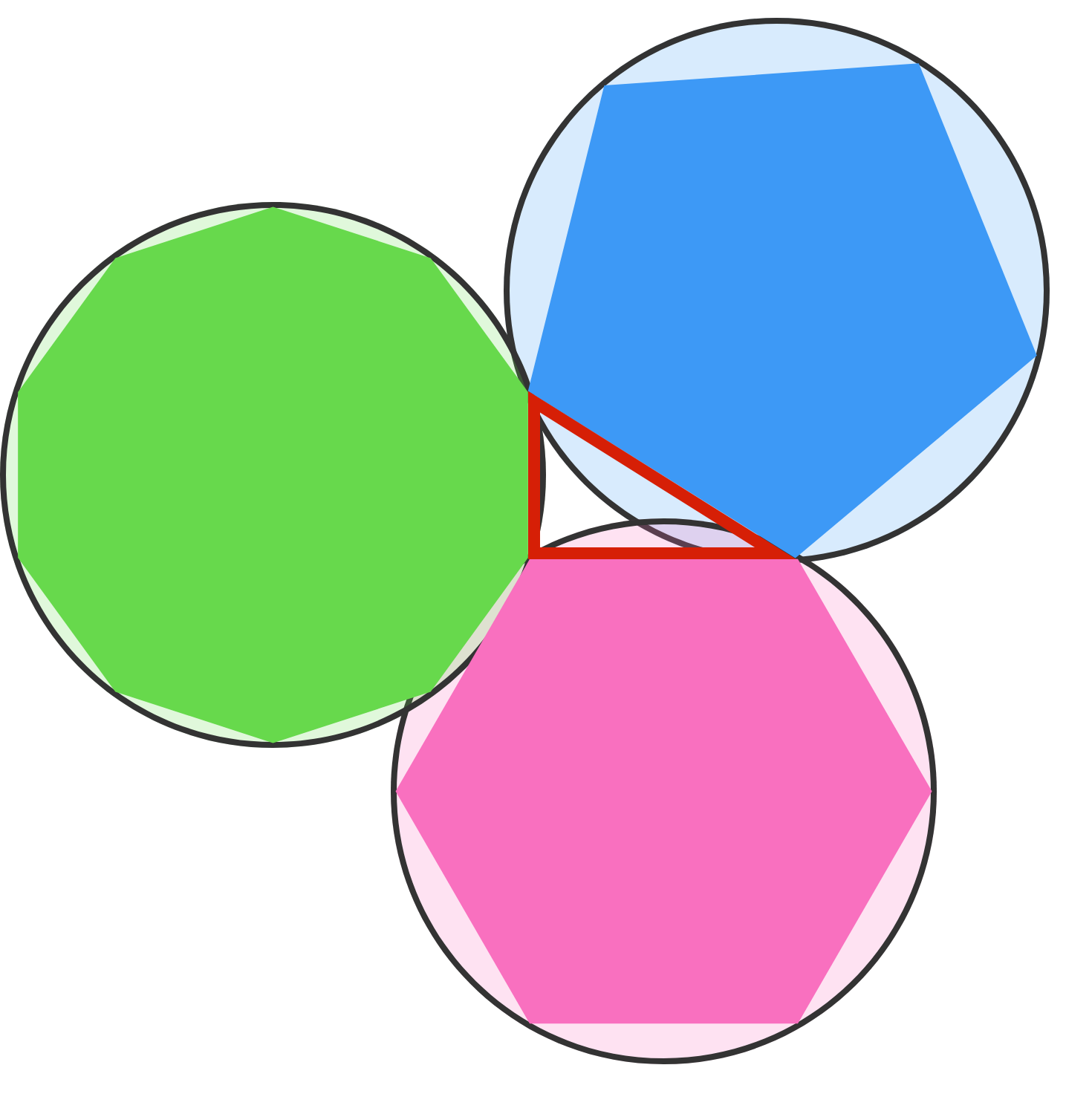# A Pentagon, Hexagon And A Decagon Walk Into A Bar

Geometry Level 2As shown in the image above, a pentagon, hexagon and decagon are inscribed in three congruent circles, and their endpoints are connected to form a triangle. If the radii of each of the circles is $1$ and the area of the triangle formed by the three polygons can be written as $\frac{\sqrt{a}-b}{c}$, where $a$, $b$ and $c$ are coprime integers, what is $a+b+c$?

×